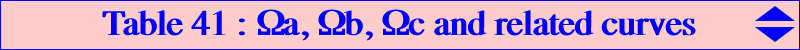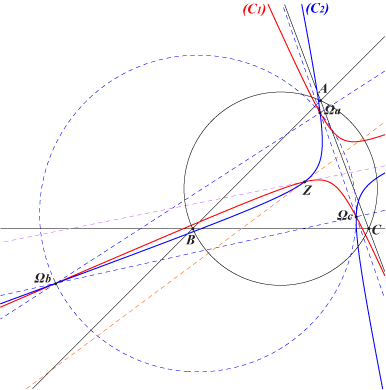The points Ωa, Ωb, Ωc are the only points for which there are infinitely many equilateral pKs with pole one of these points. They lie on a large number of curves although they are not constructible with ruler and compass only. See also CL052 and Table 18. Conics Two conicsThese three points lie on two conics (C1) and (C2) that meet at a fourth point Z, intersection of the lines X(5)X(1989) and X(6)X(30). Z = X(14836) in ETC. (C1) contains X(6), X(53), X(216) and X(1989). (C2) contains X(395), X(396), X(523) and X(3003).Cubics These points Ωa, Ωb, Ωc lie on the PΩe–transforms of all the cubics that pass through the points Ua, Ub, Uc of Table 18. The following table gives a selection of these cubics.Cubics passing through Ua, Ub, Uc Cubics passing through Ωa, Ωb, Ωc cubic centers / remark cubic centers / remark K001 see the page / circular pK K095 see the page / pK K009 see the page / nodal K486 X(6), X(53), X(2965) / nodal K080 see the page / equilateral K307 X(6), X(53), X(216), X(1249) K092 see the page / equilateral K485 X(2), X(11058), X(14537) / equilateral pK K142 see the page / equilateral - / psK(X512, X2, ?) K330 see the page / circular K487 X(2), X(6), X(67), X(187), X(11058) K331 see the page / circular X(6) / circumcubic K488 see the page X(6), X(50), X(1989) K732 see the page - / nK(X11060, X265, ?) K753 see the page / circular K277 X(6), X(37), X(216), X(395), X(396), X(1989)